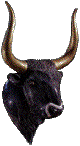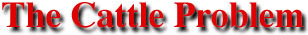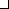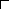S O L U T I O N . ( 1 S T . P A R T )
 Back to . . .Archimedes Home Page This section . . .Statement (English)Solution (1st part)Solution (2nd part)Statement (Greek)Computer Output (1st part)Computer Output (2nd part)

The First Part of the Cattle Problem

Let

 W = number of white bulls B = number of black bulls Y = number of yellow bulls D = number of dappled bulls w = number of white cows b = number of black cows y = number of yellow cows d = number of dappled cows
Then the first part of the problem can be stated as the following seven equations in eight unknowns:

 (1) W = (1/2 + 1/3)B + Y (the white bulls were equal to a half and a third of the black [bulls] together with the whole of the yellow [bulls]) (2) B = (1/4 + 1/5)D + Y (the black [bulls] were equal to the fourth part of the dappled [bulls] and a fifth, together with, once more, the whole of the yellow [bulls]) (3) D = (1/6 + 1/7)W + Y (the remaining bulls, the dappled, were equal to a sixth part of the white [bulls] and a seventh, together with all of the yellow [bulls]) (4) w = (1/3 + 1/4)(B + b) (The white [cows] were precisely equal to the third part and a fourth of the whole herd of the black) (5) b = (1/4 + 1/5)(D + d) (the black [cows] were equal to the fourth part once more of the dappled and with it a fifth part, when all, including the bulls, went to pasture together) (6) d = (1/5 + 1/6)(Y + y) (the dappled [cows] in four parts [i.e., in totality] were equal in number to a fifth part and a sixth of the yellow herd) (7) y = (1/6 + 1/7)(W + w) (the yellow [cows] were in number equal to a sixth part and a seventh of the white herd)

Equations (1-7) constitute a homogeneous linear system for W, B, Y, D, w, b, y, d with the following 7 x 8 coefficient matrix:6 -5 -6 0 0 0 0 00 20 -20 -9 0 0 0 0 -13 0 -42 42 0 0 0 0 0 -7 0 0 12 -7 0 0 0 0 0 -9 0 20 0 -9 0 0 -11 0 0 0 -11 30 -13 0 0 0 -13 0 42 0

Using a symbolic algebra program (Maple©, MatLab©, Mathematica©, etc.) it is easily determined that this matrix has rank seven and a one-dimensional nullspace given by

 W = 10,366,482k B = 7,460,514k Y = 4,149,387k D = 7,358,060k w = 7,206,360k b = 4,893,246k y = 5,439,213k d = 3,515,820k

where k is an arbitrary number and where the integers multiplying k have no common divisor. There are thus infinitely many possible positive integer solutions, corresponding to k = 1, 2, 3, ... . The smallest positive integer solution arises when k = 1, and so is

 W = 10,366,482 = number of white bulls B = 7,460,514 = number of black bulls Y = 4,149,387 = number of yellow bulls D = 7,358,060 = number of dappled bulls w = 7,206,360 = number of white cows b = 4,893,246 = number of black cows y = 5,439,213 = number of yellow cows d = 3,515,820 = number of dappled cows
and the total number of cattle of the Sun is 50,389,082. If you could have gotten this far, then you "wouldst not be called unskilled or ignorant of numbers, but not yet shalt thou be numbered among the wise". Only by solving the second part of the problem can you be numbered among the wise.